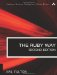# Section 5.10. Matrix Manipulation

### 5.9. Working with Rational Values

The Rational class enables us (in many cases) to work with fractional values with "infinite" precision. It helps us only when the values involved are true rational numbers (the quotient of two integers). It won't help with irrational numbers such as pi, e, or the square root of 2.

To create a rational number, we use the special method Rational (which is one of our rare capitalized method names, usually used for data conversion or initialization).

`r = Rational(1,2)   # 1/2 or 0.5 s = Rational(1,3)   # 1/3 or 0.3333... t = Rational(1,7)   # 1/7 or 0.14... u = Rational(6,2)   # "same as" 3.0 z = Rational(1,0)   # error!`

An operation on two rationals will typically be another rational:

`r+t          #  Rational(9, 14) r-t          #  Rational(5, 14) r*s          #  Rational(1, 6) r/s          #  Rational(3, 2)`

Let's look once again at our floating point inaccuracy example (see section 5.4, "Comparing Floating Point Numbers"). In the following example, we do the same thing with rationals rather than reals, and we get the "mathematically expected" results instead:

`x = Rational(1000001,1)/Rational(3,1000) y = Rational(3,1000)*x if y == 1000001.0   puts "yes"          # Now we get "yes"! else   puts "no" end`

Some operations, of course, don't always give us rationals back.

`x = Rational(9,16)    #  Rational(9, 16) Math.sqrt(x)          #  0.75 x**0.5                #  0.75 x**Rational(1,2)      #  0.75`

However, the mathn library changes some of this behavior. See section 5.12 "Using mathn."The Ruby Way, Second Edition: Solutions and Techniques in Ruby Programming (2nd Edition)
ISBN: 0672328844
EAN: 2147483647
Year: 2004
Pages: 269
Authors: Hal Fulton

Similar book on Amazon